The LOGISTIC Procedure
 Link Functions and the Corresponding Distributions

Four link functions are available in the LOGISTIC procedure. The logit function is the default. To specify a different link function, use the LINK= option in the MODEL statement. The link functions and the corresponding distributions are as follows:

• The logit function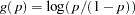is the inverse of the cumulative logistic distribution function, which is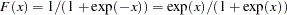• The probit (or normit) function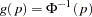is the inverse of the cumulative standard normal distribution function, which is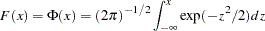Traditionally, the probit function contains the additive constant 5, but throughout PROC LOGISTIC, the terms probit and normit are used interchangeably.

• The complementary log-log function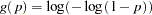is the inverse of the cumulative extreme-value function (also called the Gompertz distribution), which is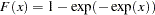• The generalized logit function extends the binary logit link to a vector of levels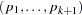by contrasting each level with a fixed level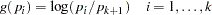The variances of the normal, logistic, and extreme-value distributions are not the same. Their respective means and variances are shown in the following table:

Distribution

Mean

Variance

Normal

0

1

Logistic

0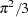Extreme-value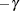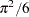Here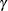is the Euler constant. In comparing parameter estimates from different link functions, you need to take into account the different scalings of the corresponding distributions and, for the complementary log-log function, a possible shift in location. For example, if the fitted probabilities are in the neighborhood of 0.1 to 0.9, then the parameter estimates from the logit link function should be about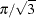larger than the estimates from the probit link function.Previous Page | Next Page | Top of Page